# Percents As Ratios How treating percents as ratios

• Slides: 13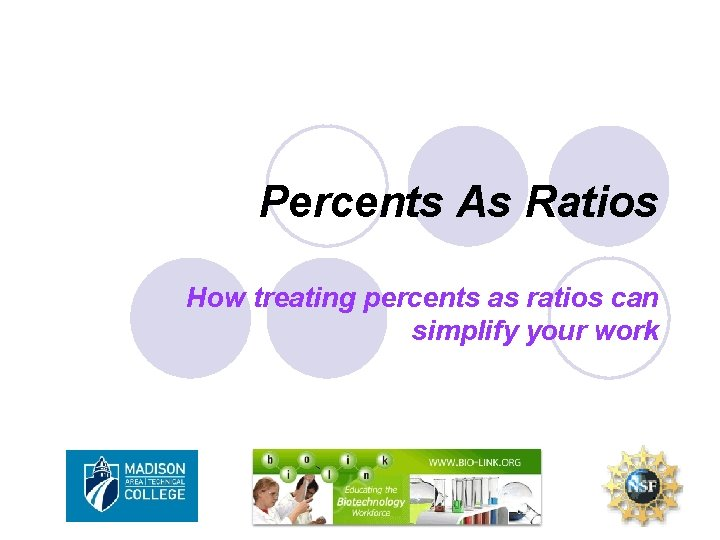Percents As Ratios How treating percents as ratios can simplify your work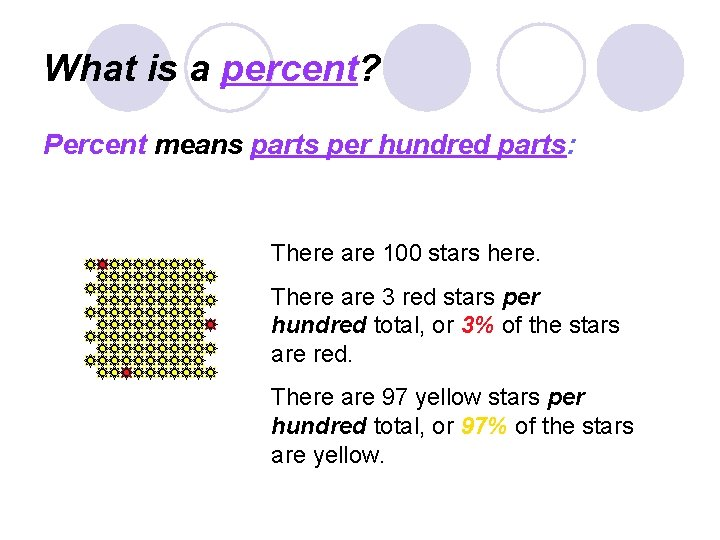What is a percent? Percent means parts per hundred parts: There are 100 stars here. There are 3 red stars per hundred total, or 3% of the stars are red. There are 97 yellow stars per hundred total, or 97% of the stars are yellow.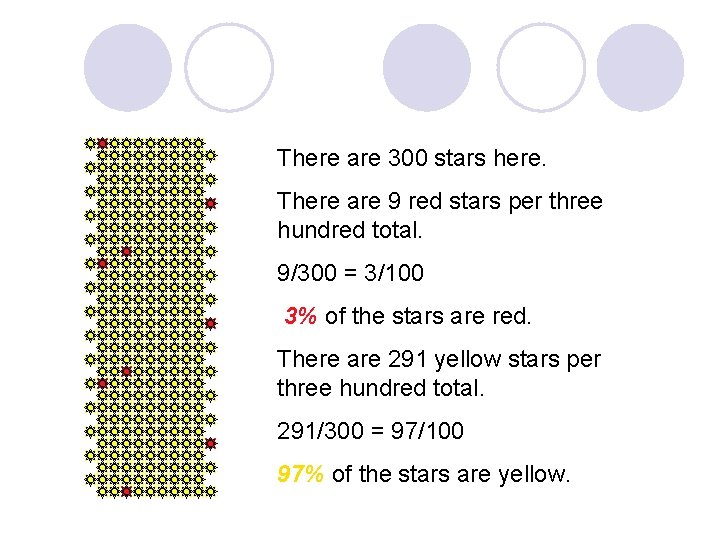There are 300 stars here. There are 9 red stars per three hundred total. 9/300 = 3/100 3% of the stars are red. There are 291 yellow stars per three hundred total. 291/300 = 97/100 97% of the stars are yellow.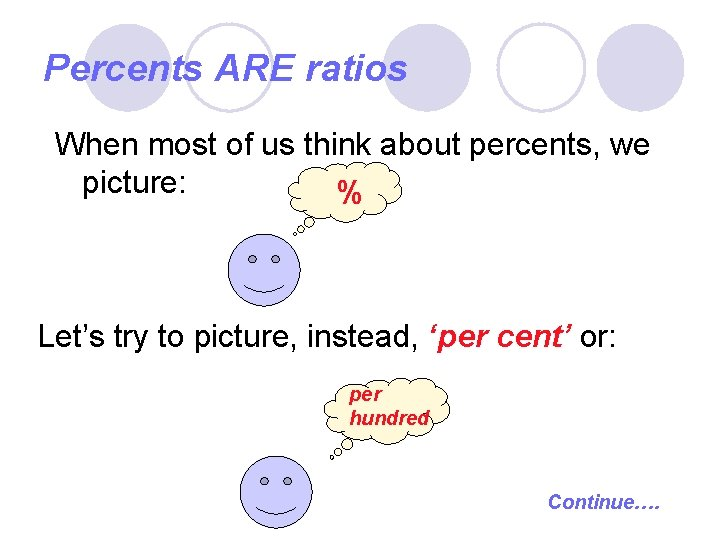Percents ARE ratios When most of us think about percents, we picture: % Let’s try to picture, instead, ‘per cent’ or: per hundred Continue….How does this help? Problem 1: Write 75% as a fraction: Easy!! “ 3/4” Who needs ratios? ? Continue….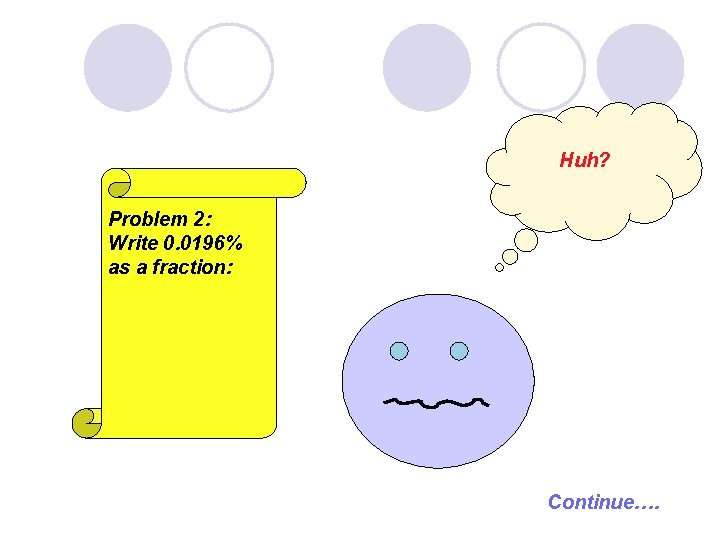Huh? Problem 2: Write 0. 0196% as a fraction: Continue….Problem 2: Write 0. 0196% as a fraction: Wait… 0. 0196% is 0. 0196 per cent Continue….Problem 2: Write 0. 0196% as a fraction: 0. 0196 per cent is: 0. 0196 per hundred or 0. 0196 100 Hurray!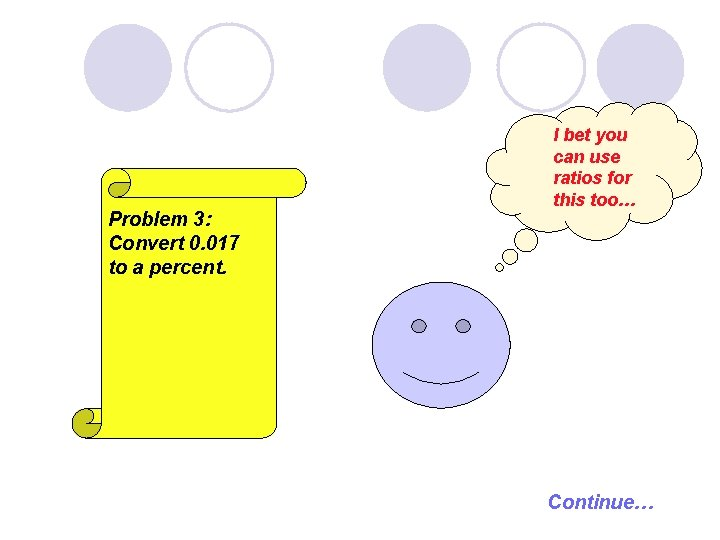Problem 3: Convert 0. 017 to a percent. I bet you can use ratios for this too… Continue…Problem 3: Convert 0. 017 to a percent. I want to know… 0. 017 = ? percent, or ? per hundred: 0. 017 = ? 100 Solve…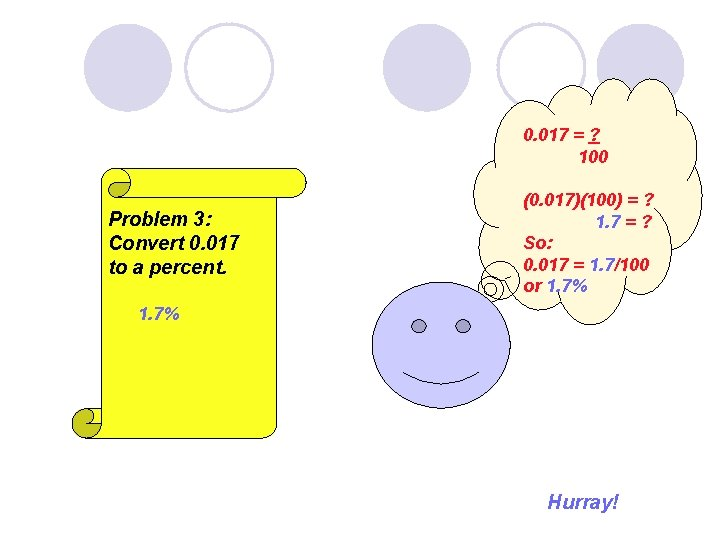0. 017 = ? 100 Problem 3: Convert 0. 017 to a percent. (0. 017)(100) = ? 1. 7 = ? So: 0. 017 = 1. 7/100 or 1. 7% Hurray!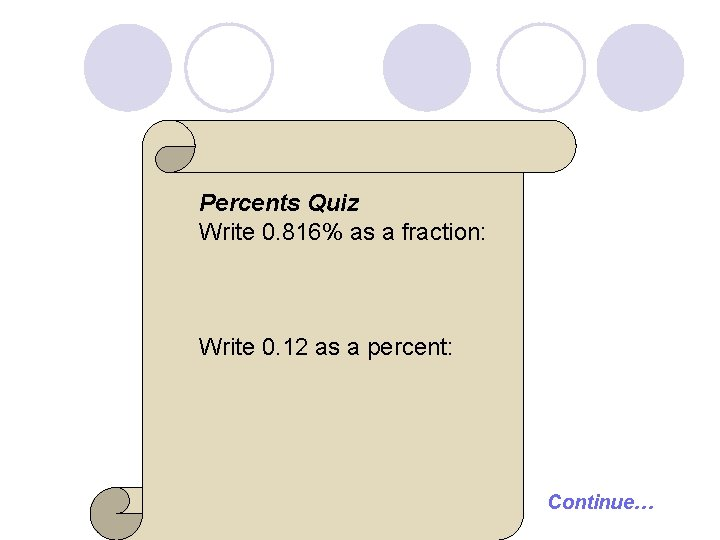Percents Quiz Write 0. 816% as a fraction: Write 0. 12 as a percent: Continue…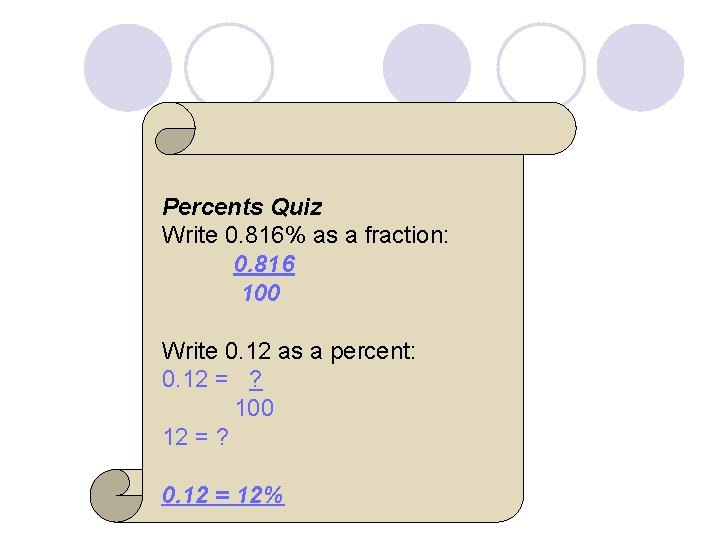Percents Quiz Write 0. 816% as a fraction: 0. 816 100 Write 0. 12 as a percent: 0. 12 = ? 100 12 = ? 0. 12 = 12%